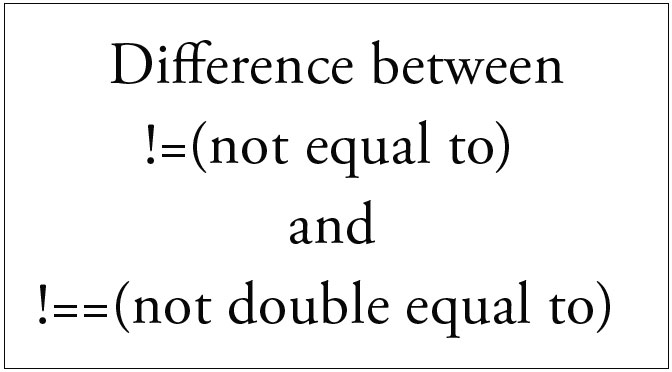# Difference between !=(not equal to) and !==(not double equal to) in phpHopefully, you can think that !== operator as being more ‘strict’ than the != operator.

This is because the !== operator will say that the two operands being compared are not equal only if the type of the two operands are the same, but their values are not equal.

This is desirable behaviour because the strpos function can return a 0 if the string being searched contains the substring as the very first element.The 0 would represent the 0th index of the larger string, meaning the first position in that string. This means that the check being performed will be to see if 0 is not equal to false. But the problem is that 0 is also considered as the integer equivalent of the boolean ‘false’ in PHP, which means that the statement ‘0 != false’ will be considered false, because 0 is equal to false in PHP.

But, if we run ‘0 !== false’ instead, then that statement will be considered to be true, because it just adds the additional check to see if 0 and false are of the same type. Since 0 is an integer and false is a boolean, clearly they are not equal so comparing the 0 and false for inequality returns true unlike the ‘0 != false’ check, which returns false.

Confusing?

Let’s take an example

[php]
<?php

if (strpos(\$string,’ScriptArticle’) != false) {
echo ‘I found ScriptArticle!’;
}

?>
[/php]

As the ‘ScriptArticle’ should found on the string and it should print ‘I found ScriptArticle!’, but as it is on 0th index so it considered as false and it will not print anything at all.

But if you use ‘!==’ instead of ‘!=’ then it will compared the types also and 0 is integer and false is boolean so the condition will satisfy and print the statement inside the if.